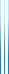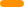# PublicationsPapers

## Exponential lower bounds for $\mathsf{AC^0}$-Frege imply superpolynomial Frege lower bounds

Yuval Filmus, Toniann Pitassi and Rahul Santhanam
ICALP 2011, ToCT

We give a general transformation which turns polynomial-size Frege proofs to subexponential-size $\mathsf{AC^0}$-Frege proofs. This indicates that proving exponential lower bounds for $\mathsf{AC^0}$-Frege is hard, since it is a longstanding open problem to probe super-polynomial lower bounds for Frege. Our construction is optimal for tree-like proofs.

As a consequence of our main result, we are able to shed some light on the question of weak automatizability for bounded-depth Frege systems. First, we present a simpler proof of the results of Bonet et al. showing that under cryptographic assumptions, bounded-depth Frege proofs are not weakly automatizable. Second, we show that because our proof is more general, under the right cryptographic assumptions it could resolve the weak automatizatbility question for lower depth Frege systems.

The proceedings version contains several small mistakes, corrected in the preprint version. These mistakes slightly affect the constants in the main theorem.

## BibTeX

@inproceedings{FPS2011,
author = {Yuval Filmus and Toniann Pitassi and Rahul Santhanam},
title = {Exponential lower bounds for {AC$^0$}-{F}rege imply
superpolynomial {F}rege lower bounds},
booktitle = {The 38th International Colloquium on Automata,
Languages and Programming ({ICALP} 2011)},
year = {2011},
pages = {618--629}
}

@article{FPS2015,
author = {Yuval Filmus and Toniann Pitassi and Rahul Santhanam},
title = {Exponential lower bounds for {AC$^0$}-{F}rege imply
superpolynomial {F}rege lower bounds},
journal = {ACM Trans. Comput. Th.},
volume = {7},
number = {2},
year = {2015},
pages = {article 5}
}copy to clipboard